NEET  >  31 Year NEET Previous Year Questions: Motion in a Plane - 2

# 31 Year NEET Previous Year Questions: Motion in a Plane - 2

Test Description

## 30 Questions MCQ Test Physics Class 11 | 31 Year NEET Previous Year Questions: Motion in a Plane - 2

31 Year NEET Previous Year Questions: Motion in a Plane - 2 for NEET 2023 is part of Physics Class 11 preparation. The 31 Year NEET Previous Year Questions: Motion in a Plane - 2 questions and answers have been prepared according to the NEET exam syllabus.The 31 Year NEET Previous Year Questions: Motion in a Plane - 2 MCQs are made for NEET 2023 Exam. Find important definitions, questions, notes, meanings, examples, exercises, MCQs and online tests for 31 Year NEET Previous Year Questions: Motion in a Plane - 2 below.
Solutions of 31 Year NEET Previous Year Questions: Motion in a Plane - 2 questions in English are available as part of our Physics Class 11 for NEET & 31 Year NEET Previous Year Questions: Motion in a Plane - 2 solutions in Hindi for Physics Class 11 course. Download more important topics, notes, lectures and mock test series for NEET Exam by signing up for free. Attempt 31 Year NEET Previous Year Questions: Motion in a Plane - 2 | 30 questions in 60 minutes | Mock test for NEET preparation | Free important questions MCQ to study Physics Class 11 for NEET Exam | Download free PDF with solutions
 1 Crore+ students have signed up on EduRev. Have you?
31 Year NEET Previous Year Questions: Motion in a Plane - 2 - Question 1

### The magnitudes of vectors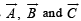are 3, 4 and 5 units respectively. If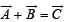, thenthe angle between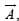and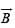is

Detailed Solution for 31 Year NEET Previous Year Questions: Motion in a Plane - 2 - Question 1

Given,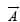= 3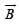= 4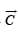= 5

We have a relation,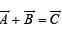Squaring both side, we get

⇒ (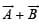) = (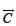)2

⇒(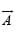)2+ (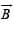)2 + 2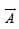Cosθ = (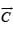)2

⇒ (3)2 + (4)2 + 2.3.4 Cosθ = (5)2

⇒ 9 + 16 + 24 Cosθ = 25

⇒ 24 Cosθ = 25

⇒ 24Cosθ = 25 - 25

⇒ θ = Cos-1 (0)

⇒ θ = 90°

i.e π/2

31 Year NEET Previous Year Questions: Motion in a Plane - 2 - Question 2

### The angle between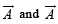is θ.  The value of the triple product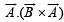is 

Detailed Solution for 31 Year NEET Previous Year Questions: Motion in a Plane - 2 - Question 2

Note that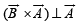. Hence their dot product is zero.

31 Year NEET Previous Year Questions: Motion in a Plane - 2 - Question 3

### A bus is moving on a straight road towards north with a uniform speed of 50 km/hour turns through 90°. If the speed remains unchanged after turning, the increase in the velocity of bus in the turning process is 

Detailed Solution for 31 Year NEET Previous Year Questions: Motion in a Plane - 2 - Question 3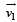= 50 km h-1 due North;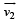= 50 km h-1 due West. Angle between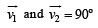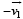= 50 km h-1  due South

∴ Change in velocity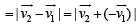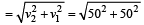= 70.7 km/h

The direction of this change in velocity is in South-West.

31 Year NEET Previous Year Questions: Motion in a Plane - 2 - Question 4

The maximum range of a gun of horizontal terrain is 16 km. If g = 10 ms–2, then muzzle velocity of a shell must be 

Detailed Solution for 31 Year NEET Previous Year Questions: Motion in a Plane - 2 - Question 4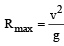= 16000 [16km = 16000m]

or  v = (16000g )½ = (16000 x10)½

= 400 ms –1

31 Year NEET Previous Year Questions: Motion in a Plane - 2 - Question 5

Two bodies of same mass are projected with the same velocity at an angle 30° and 60° respectively. The ratio of their horizontal ranges will be

Detailed Solution for 31 Year NEET Previous Year Questions: Motion in a Plane - 2 - Question 5

Horizontal range is same when angle of projection is θ or (90° – θ).

31 Year NEET Previous Year Questions: Motion in a Plane - 2 - Question 6

An electricfan has blades of length 30cm measured from the axis of rotation. If the fan is rotating at 120 rpm, the acceleration of a point on the tip of the blade is 

Detailed Solution for 31 Year NEET Previous Year Questions: Motion in a Plane - 2 - Question 6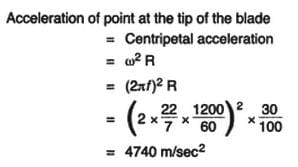31 Year NEET Previous Year Questions: Motion in a Plane - 2 - Question 7

When milk is churned, cream gets separated due to

Detailed Solution for 31 Year NEET Previous Year Questions: Motion in a Plane - 2 - Question 7

Cream gets separated from a churned milk due to centrifugal force.

31 Year NEET Previous Year Questions: Motion in a Plane - 2 - Question 8

The resultant of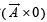will be equal to 

Detailed Solution for 31 Year NEET Previous Year Questions: Motion in a Plane - 2 - Question 8

When a vector is multiplied with a scalar, the result is a vector.

31 Year NEET Previous Year Questions: Motion in a Plane - 2 - Question 9

If a body  A  of mass  M  is thrown with velocity v  at an angle of 30° to the horizontal and another body  B  of the  same mass is thrown with the same speed at an angle of 60° to the horizontal, the ratio of horizontal range of A to B will be 

Detailed Solution for 31 Year NEET Previous Year Questions: Motion in a Plane - 2 - Question 9

Horizontal range is same wh en an gle of projection with the horizonatal is θ and (90° – θ).

31 Year NEET Previous Year Questions: Motion in a Plane - 2 - Question 10

A boat is sent across a river with a velocity of 8 km h–1. If the resultant velocity of boat is 10 km h–1 , then the velocity of the river is 

Detailed Solution for 31 Year NEET Previous Year Questions: Motion in a Plane - 2 - Question 10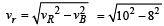= 6 km h –111.

31 Year NEET Previous Year Questions: Motion in a Plane - 2 - Question 11

A body constrained to move in y-direction, is subjected to a force given  by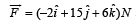. What is the workdone by this force in moving the body through a distance of 10m along y-axis ? 

Detailed Solution for 31 Year NEET Previous Year Questions: Motion in a Plane - 2 - Question 11

Since displacement is along the y-direction, hence displacement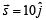Work done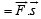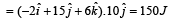31 Year NEET Previous Year Questions: Motion in a Plane - 2 - Question 12

When a body moves with a constant speed along a circle

Detailed Solution for 31 Year NEET Previous Year Questions: Motion in a Plane - 2 - Question 12

On circular motion, the force acts along the radius and displacement at a location is perpendicular to the radius i.e., θ = 90° As work done =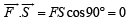31 Year NEET Previous Year Questions: Motion in a Plane - 2 - Question 13

Th e position vector of a par ticle is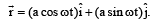. The velocity of the particle is 

Detailed Solution for 31 Year NEET Previous Year Questions: Motion in a Plane - 2 - Question 13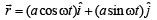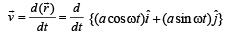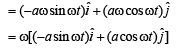Slope of position vector =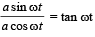& slope of velocity vector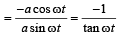∴  velocity is perpendicular to the displacement.

31 Year NEET Previous Year Questions: Motion in a Plane - 2 - Question 14

Which of the following is not a vector quantity?

Detailed Solution for 31 Year NEET Previous Year Questions: Motion in a Plane - 2 - Question 14

A vector quantity has both magnitude and direction. In the given options, speed has only magnitude, therefore, it is non- vector or scalar quantity.

31 Year NEET Previous Year Questions: Motion in a Plane - 2 - Question 15

A bullet is fired from a gun with a speed of 1000 m/s in order to hit a target 100 m away. At what height above the target should the gun be aimed? (The resistance of air is negligible and g = 10 m/s2) 

Detailed Solution for 31 Year NEET Previous Year Questions: Motion in a Plane - 2 - Question 15

Speed of the bullet (v) = 1000 m/s and horizontal distance of the target (s) = 100 m.
Time taken to cover the horizontal distance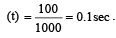During this time, the bullet will fall down vertically due to gravitational acceleration.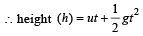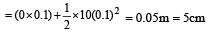31 Year NEET Previous Year Questions: Motion in a Plane - 2 - Question 16

A body is whirled in a horizontal circle of radius 20 cm. It has an angular velocity of 10 rad/s.What is its linear velocity at any point on circular path

Detailed Solution for 31 Year NEET Previous Year Questions: Motion in a Plane - 2 - Question 16

Radius of circular path = 20 cm =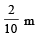Angular speed of body = 10 rad/s

Linear velocity = radius × Angular speed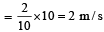31 Year NEET Previous Year Questions: Motion in a Plane - 2 - Question 17

A ball whose kinetic energy is E, is thrown at an angle of 45º with the horizontal. Its kinetic energy at the highest point of its flight will be 

Detailed Solution for 31 Year NEET Previous Year Questions: Motion in a Plane - 2 - Question 17

Kinetic energy is E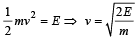Velocity at highest point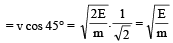[∴ at the highest point there is only horizontal velocity]

K.E. at highest point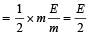31 Year NEET Previous Year Questions: Motion in a Plane - 2 - Question 18

Find the torque of a force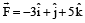acting at the poin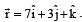. 

Detailed Solution for 31 Year NEET Previous Year Questions: Motion in a Plane - 2 - Question 18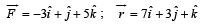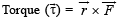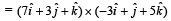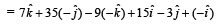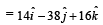31 Year NEET Previous Year Questions: Motion in a Plane - 2 - Question 19

Two particles A an d B are con nected by a rigid rod AB. The rod slides along perpendicular rails as shown here. The velocity of A to the left is 10 m/s. What is the velocity of B when angle α = 60º?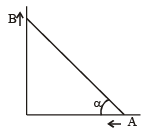Detailed Solution for 31 Year NEET Previous Year Questions: Motion in a Plane - 2 - Question 19

Let after 1 sec angle become 60°. When the end A moves by 10 m left, the end B moves upward by BB' =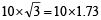= 17.3 m/s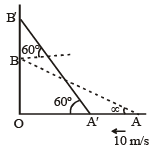31 Year NEET Previous Year Questions: Motion in a Plane - 2 - Question 20

A ball of mass 0.25 kg attached to the end of a string of length 1.96 m is moving in a horizontal circle. The string will break if the tension is more than 25 N. What is the maximum speed with which the ball can be moved? 

Detailed Solution for 31 Year NEET Previous Year Questions: Motion in a Plane - 2 - Question 20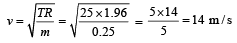31 Year NEET Previous Year Questions: Motion in a Plane - 2 - Question 21

A 500 kg car takes a round turn of radius 50 m with a velocity of 36 km/h. The centripetal force is

Detailed Solution for 31 Year NEET Previous Year Questions: Motion in a Plane - 2 - Question 21

V =36km/h=10m/s

Centripetal force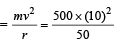= 1000 N

31 Year NEET Previous Year Questions: Motion in a Plane - 2 - Question 22

What is the linear velocity if angular velocity vect or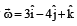and position vector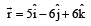Detailed Solution for 31 Year NEET Previous Year Questions: Motion in a Plane - 2 - Question 22

As we know that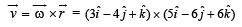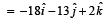31 Year NEET Previous Year Questions: Motion in a Plane - 2 - Question 23

The angle between two vectors of magnitude 12 and 18 units when their resultant is 24 units, is

Detailed Solution for 31 Year NEET Previous Year Questions: Motion in a Plane - 2 - Question 23

We know that, R2 = A2 + B2 + 2 AB cosθ
(24)2 = (12)2 + (18)2 + 2(12)(18) cos θ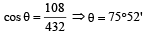31 Year NEET Previous Year Questions: Motion in a Plane - 2 - Question 24

A body of mass 0.4 kg is whi rled in a vertical circle making 2 rev/sec. If the radius of the circle is 1.2 m, then tension in the string when the body is at th e top of the circle, is 

Detailed Solution for 31 Year NEET Previous Year Questions: Motion in a Plane - 2 - Question 24

Given : Mass (m) = 0.4 kg Its frequency (n) = 2 rev/sec Radius (r) =1.2 m.

We know that linear velocity of the body (v) = ωr = (2πn)r

= 2 × 3.14 × 1.2 × 2 = 15.08 m/s.

Therefore, tension in the string when the body is at the top of the circle (T)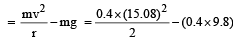= 45.78 - 3.92 = 41.56 N

31 Year NEET Previous Year Questions: Motion in a Plane - 2 - Question 25

A stone tied with a string, is rotated in a vertical circle. The minimum speed with which the string has to be rotated 

Detailed Solution for 31 Year NEET Previous Year Questions: Motion in a Plane - 2 - Question 25

Minimum speed with which the string is rotating in a vertical circle (v) =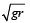The minimum speed of stone is independent of mass of stone.

31 Year NEET Previous Year Questions: Motion in a Plane - 2 - Question 26

A person swims in a river aiming to reach exactly opposite point on the bank of a river. His speed of swimming is 0.5 m/s at an angle 120º with the direction of flow of water. The speed of water in stream is 

Detailed Solution for 31 Year NEET Previous Year Questions: Motion in a Plane - 2 - Question 26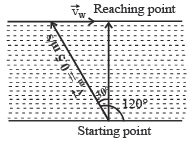Velocity of person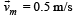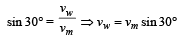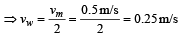31 Year NEET Previous Year Questions: Motion in a Plane - 2 - Question 27

If a unit vector is represented by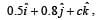, the value of c is 

Detailed Solution for 31 Year NEET Previous Year Questions: Motion in a Plane - 2 - Question 27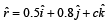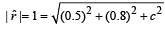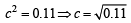31 Year NEET Previous Year Questions: Motion in a Plane - 2 - Question 28

Two projectiles ar e fir ed from t he same point with the same speed at angles of projection 60º and 30º respectively. Which one of the following is true? 

Detailed Solution for 31 Year NEET Previous Year Questions: Motion in a Plane - 2 - Question 28

Given, u1 = u2 = u, θ1 = 60º, θ2 = 30º In 1st case, we know that range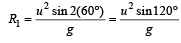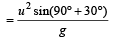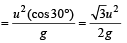In 2nd case, when θ2 = 30º, then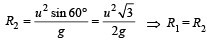[we get same value of ranges].

31 Year NEET Previous Year Questions: Motion in a Plane - 2 - Question 29

A small sphere is attach ed to a cord and rotates in a vertical circle about a point O. If the average speed of the sphere is increased, the cord is most likely to break at the orientation when the mass is at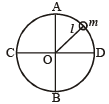Detailed Solution for 31 Year NEET Previous Year Questions: Motion in a Plane - 2 - Question 29

In the case of a body describing a vertical circle,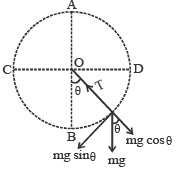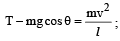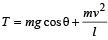Tension is maximum when cosθ = +1 and velocity is maximum Both conditions are satisfied at θ = 0º (i.e. at lowest point B]

31 Year NEET Previous Year Questions: Motion in a Plane - 2 - Question 30

A particle moves with a velocity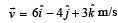under the in fluence of a constant force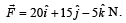The instantaneous power applied to the particle is 

Detailed Solution for 31 Year NEET Previous Year Questions: Motion in a Plane - 2 - Question 30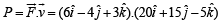= 6 × 20 – 4 × 15 –3 ×5 = 45 J/s

## Physics Class 11

127 videos|464 docs|210 tests
 Use Code STAYHOME200 and get INR 200 additional OFF Use Coupon Code
Information about 31 Year NEET Previous Year Questions: Motion in a Plane - 2 Page
In this test you can find the Exam questions for 31 Year NEET Previous Year Questions: Motion in a Plane - 2 solved & explained in the simplest way possible. Besides giving Questions and answers for 31 Year NEET Previous Year Questions: Motion in a Plane - 2, EduRev gives you an ample number of Online tests for practice

## Physics Class 11

127 videos|464 docs|210 tests

### How to Prepare for NEET

Read our guide to prepare for NEET which is created by Toppers & the best Teachers